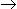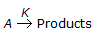# Chemical Engineering - Chemical Reaction Engineering

### Exercise :: Chemical Reaction Engineering - Section 7

41.

The importance of diffusion in a catalyst are increased by

 A. large catalyst particle size. B. an active surface of the catalyst. C. small pore diameter. D. all (a), (b) and (c).

Explanation:

No answer description available for this question. Let us discuss.

42.

A back mix reactor is

 A. suitable for gas phase reactions. B. ideal at very low conversion. C. same as plug flow reactor (PFR). D. same as ideal stirred tank reactor.

Explanation:

No answer description available for this question. Let us discuss.

43.

Which one is the rate controlling step in a solid-gas non-catalytic reaction occurring at very high temperature?

 A. Pore diffusion B. Film diffusion C. Ash layer diffusion D. Chemical reaction

Explanation:

No answer description available for this question. Let us discuss.

44.

The rate of the heterogenous catalytic reaction A(g) + B(g)C(g) is given by -rA = k.KA.pA.PB/(1 + KA.PA + Kc.pc), where KA and Kc are the adsorption equilibrium constants. The rate controlling step for this reaction is

 A. absorption of A. B. surface reaction between absorbed A and absorbed B. C. surface reaction between absorbed A and B in the gas phase. D. surface reaction between A in the gas phase and absorbed B.

Explanation:

No answer description available for this question. Let us discuss.

45.

For a __________ order chemical reaction,, the half life period is independent of the initial concentration of the reactant A.

 A. zero B. first C. second D. third